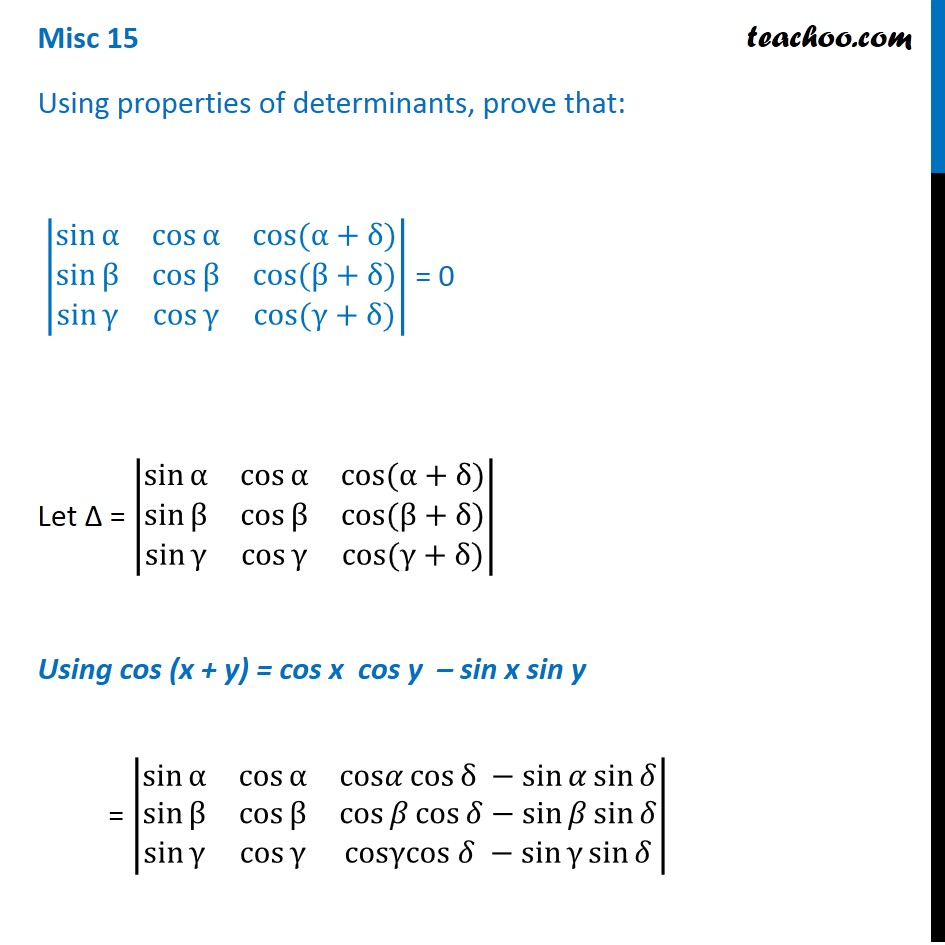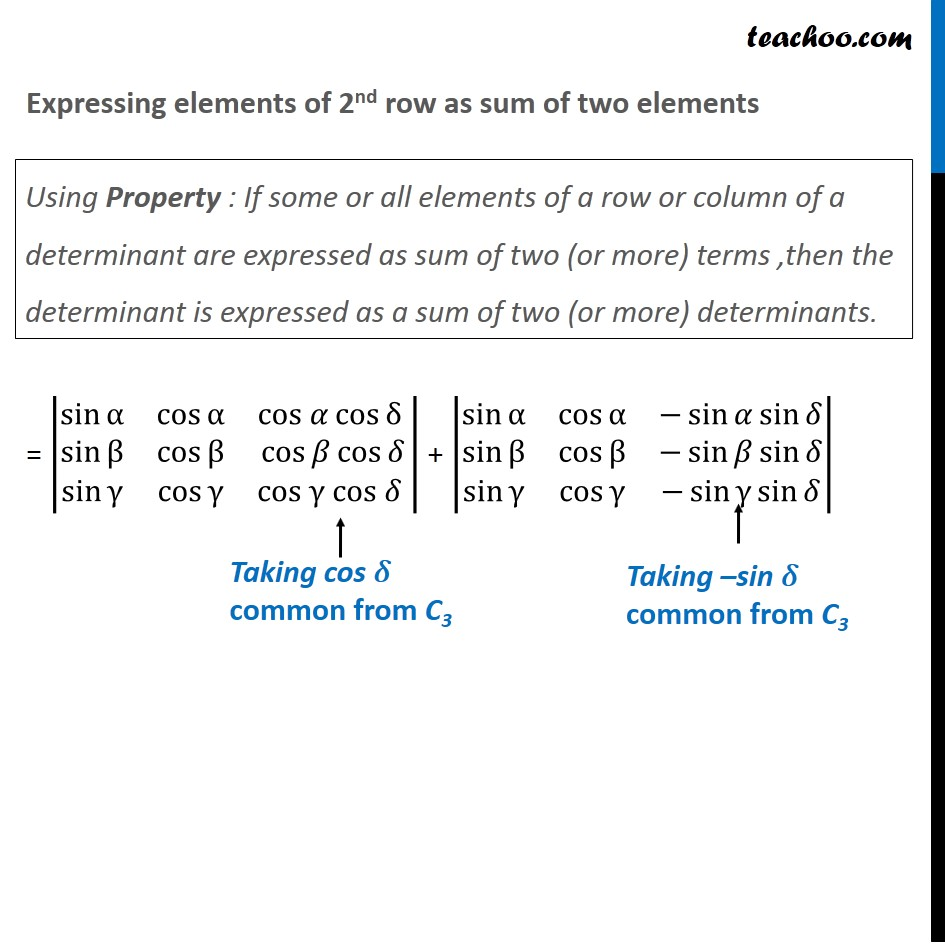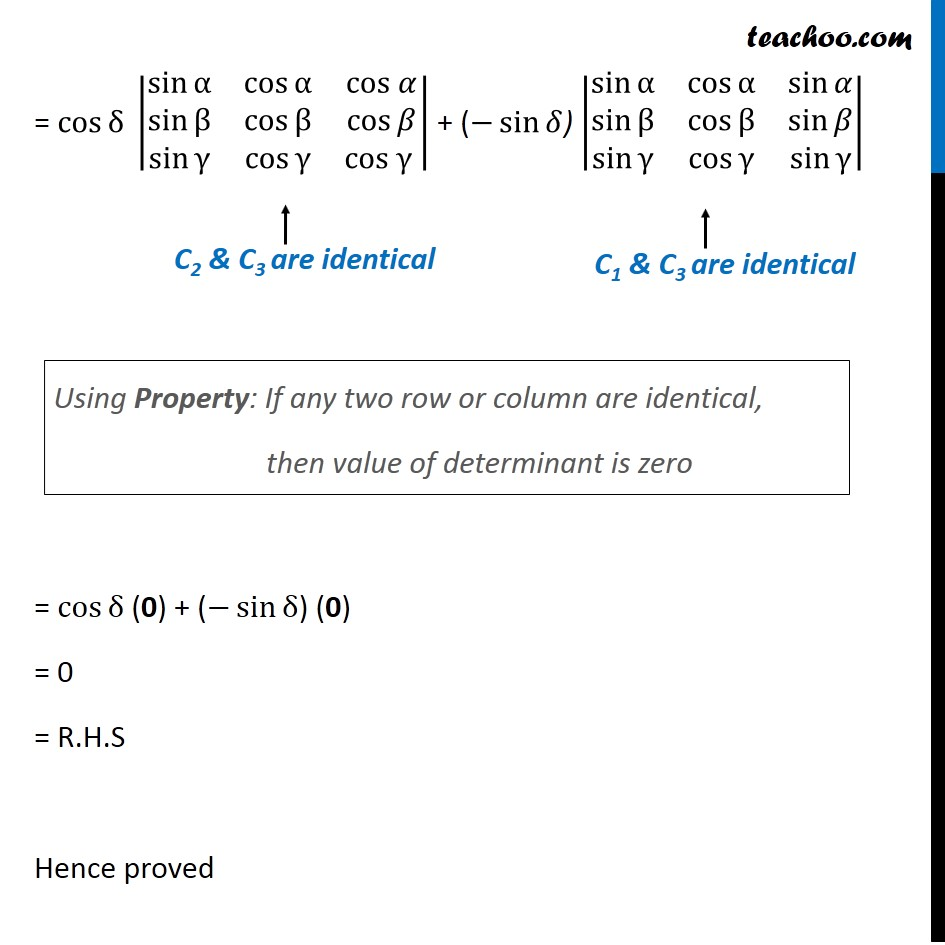Miscellaneous

Chapter 4 Class 12 Determinants
Serial order wiseLearn in your speed, with individual attention - Teachoo Maths 1-on-1 Class

### Transcript

Question 9 Using properties of determinants, prove that: |■8(sin⁡α&cos⁡α&cos⁡〖(α+δ)〗@sin⁡β&cos⁡β&cos⁡〖(β+δ)〗@sin⁡γ&cos⁡γ&cos⁡〖(γ+δ)〗 )| = 0 Let ∆ = |■8(sin⁡α&cos⁡α&cos⁡〖(α+δ)〗@sin⁡β&cos⁡β&cos⁡〖(β+δ)〗@sin⁡γ&cos⁡γ&cos⁡〖(γ+δ)〗 )| Using cos (x + y) = cos x cos y – sin x sin y = |■8(sin⁡α&cos⁡α&cos𝛼 cos⁡〖δ −sin⁡〖𝛼 sin⁡𝛿 〗 〗@sin⁡β&cos⁡β&cos⁡𝛽 cos⁡〖𝛿−sin⁡〖𝛽 sin⁡𝛿 〗 〗@sin⁡γ&cos⁡γ&cosγcos 𝛿 −sin⁡〖γ sin⁡𝛿 〗 )| Expressing elements of 2nd row as sum of two elements = |■8(sin⁡α&cos⁡α&cos 𝛼 cos⁡〖δ 〗@sin⁡β&cos⁡β&cos⁡𝛽 cos⁡𝛿@sin⁡γ&cos⁡γ&cos γ cos 𝛿 )| + |■8(sin⁡α&cos⁡α&−sin⁡〖𝛼 sin⁡𝛿 〗@sin⁡β&cos⁡β&−sin⁡〖𝛽 sin⁡𝛿 〗@sin⁡γ&cos⁡γ&−sin⁡〖γ sin⁡𝛿 〗 )| Using Property : If some or all elements of a row or column of a determinant are expressed as sum of two (or more) terms ,then the determinant is expressed as a sum of two (or more) determinants. Taking cos 𝛿 common from C3 = cos⁡〖δ 〗 |■8(sin⁡α&cos⁡α&cos 𝛼@sin⁡β&cos⁡β&cos⁡𝛽@sin⁡γ&cos⁡γ&cos γ )| + (−sin⁡𝛿) |■8(sin⁡α&cos⁡α&sin⁡𝛼@sin⁡β&cos⁡β&sin⁡𝛽@sin⁡γ&cos⁡γ&sin⁡γ )| = cos⁡〖δ 〗(0) + (−sin⁡δ) (0) = 0 = R.H.S Hence proved Using Property: If any two row or column are identical, then value of determinant is zero